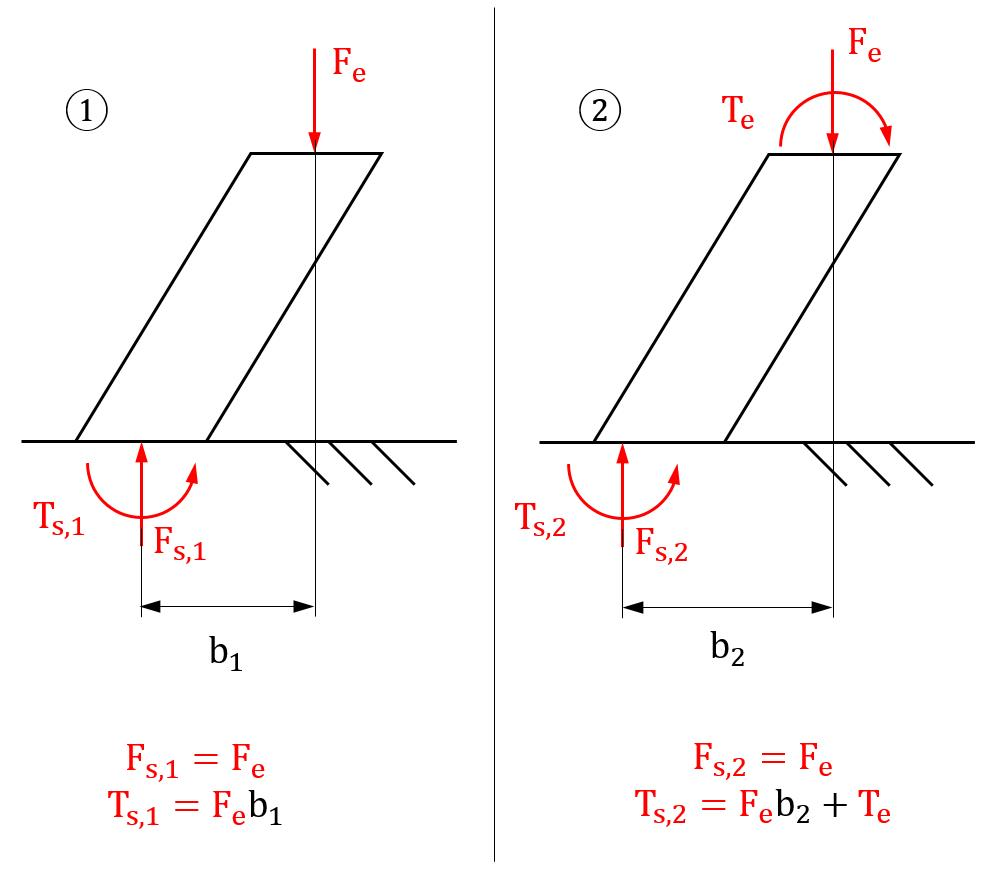## General Mechanical

•ZioMott
Subscriber
```I have an assembly on which two loads are applied in subsequent load steps:

1.  An axial force F_e;
2.  An external torque T_e.

One component of the assembly is fixed to the ground on one of its faces (with a "Fixed Support").
ANSYS allows calculating the reaction force F_{s,i} and torque T_{s,i} acting on the support at load step i. Intuitively, I expected to find T_{s,2} - T_{s,1}=T_e, but that is not the case in my simulation.
However, I also found out that F_{s,2}  != F_{s,1}; since the reaction force is not the same in the two load steps, this should also contribute to the change in the torque. I can take into account the change in F_s, but I need to know the application point of this reaction force on the supported face, for both load steps. ANSYS provides the option of calculating the total reaction torque T_s with respect to the origin of a given coordinate system, but I cannot find a way to extract information on the load application point for F_{s,1} and F_{s,2}.
My questions are:

1.  Is the reasoning above correct?
2.  How can I obtain the application point for F_s?

I am working in ANSYS 2019 R1.
•jj77
Subscriber

The reactions at the support are/should always be equal and opposite to the applied loads - simple just equilibrium.

•ZioMott
Subscriber

Hello, thanks for the prompt answer; unfortunately, there seems to be a misunderstanding.

I apologize for not being clearer in my statement of the problem; I have drawn an extremely simplified schematic that (I hope) will be clearer.

Consider the image below, where the parallelogram shape represents a loaded body that is in contact with a fixed surface. I have two load steps in which a force F_e and a torqueT_e are applied in succession. I wanted to verify that T_{s,2} - T_{s,1}=T_e, but that does not seem to be the case in my simulation; this however could be explained if the application point of the reaction force F_s has changed between the two load steps.

I am looking for a way to find the application point of the reaction force F_s. Any help is greatly appreciated.•jj77
Subscriber

This is a moment not a torque.

Anyhow to get the reactions use the Probe Force/Moment Reaction.